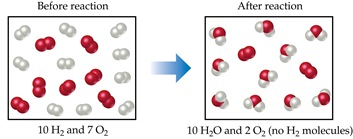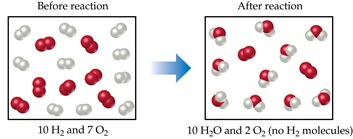# Problem: Because H2 is completely consumed, it is the limiting reactant. Because some O2 is left over after the reaction is complete, it is the excess reactant. The amount of H2O formed depends on the amount of limiting reactant, H2. If the amount of H2 is doubled, how many moles of H2O would have formed?

###### FREE Expert Solution2 H2 + O2  →  2 H2O

90% (303 ratings)###### Problem DetailsBecause H2 is completely consumed, it is the limiting reactant. Because some O2 is left over after the reaction is complete, it is the excess reactant. The amount of H2O formed depends on the amount of limiting reactant, H2. If the amount of H2 is doubled, how many moles of H2O would have formed?